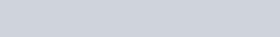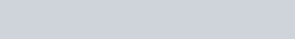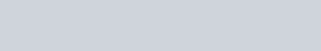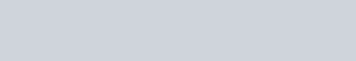Chemical Bonding Class 11 Questions with Answers-Important for Exams
Get Chemical Bonding important questions for class 11 exams. View the Important Question bank for Class 11& 12 Chemistry syllabus. These important questions will play significant role in clearing concepts of Chemistry. These questions are designed keeping NCERT class 11 chemical bonding topic in mind for various exams. The questions are updated with respect to upcoming Board exams. You will get here all the important questions for class 11 chemistry chapters. Learn all the concepts of Chemical Bonding class 11 chemistry with these questions and answers. Also, you can get notes of eact topic of Chemistry class 11 & 12 o our website.Click Here for Detailed Chapter-wise Notes of Chemistry for Class 11th, JEE & NEET. You can access free study material for all three subject’s Physics, Chemistry and Mathematics.Click Here for Detailed Notes of any chapter. eSaral provides you complete edge to prepare for Board and Competitive Exams like JEE, NEET, BITSAT, etc. We have transformed classroom in such a way that a student can study anytime anywhere. With the help of AI we have made the learning Personalized, adaptive and accessible for each and every one. Visit eSaral Website to download or view free study material for JEE & NEET. Also get to know about the strategies to Crack Exam in limited time period.
Q. Arrange the following molecules in increasing order of ionic character of their bonds $L i F, K_{2} O, N_{2}, S O_{2}, C I F_{3}$

Download eSaral App for Video Lectures, Complete Revision, Study Material and much more...

Sol. $\mathrm{N}_{2}<\mathrm{SO}_{2}<\mathrm{ClF}_{3}<\mathrm{K}_{2} \mathrm{O}<\mathrm{LiF}$

Q. Arrange the following bonds in increasing order of ionic character $C-H, F-H, B r-H, N a-I, K-F, L i-C l$

Download eSaral App for Video Lectures, Complete Revision, Study Material and much more...

Sol. $\mathrm{C}-\mathrm{H}<\mathrm{Br}-\mathrm{H}<\mathrm{Li}-\mathrm{Cl}<\mathrm{Na}-\mathrm{I}<\mathrm{K}-\mathrm{F}$

Q. Write Lewis dot symbols for the following elements:$M g, N a, B, O, N, B r, B e$ [NCERT]

Download eSaral App for Video Lectures, Complete Revision, Study Material and much more...

Sol.Q. Write Lewis symbol for the following atoms and ions :$S$ and $S^{2-}, \quad A l$ and $A I^{3+}, \quad H$ and $H^{-}$ [NCERT]

Download eSaral App for Video Lectures, Complete Revision, Study Material and much more...

Sol.Q. Write Lewis dot symbols for the following ions:$L i^{+}, C l^{-}, O^{2-}, M g^{2+}$ and $N^{3-} . \quad$ [NCERT]

Download eSaral App for Video Lectures, Complete Revision, Study Material and much more...

Sol.Q. Why is $\sigma$ -bond stronger than $\pi$ -bond?

Download eSaral App for Video Lectures, Complete Revision, Study Material and much more...

Sol. It is because extent of overlapping is more in $\sigma$ -bond than $\pi$ bond.

Q. What is the total number of sigma and $p i$ bonds in the following molecules:(i) $\mathrm{C}_{2} \mathrm{H}_{2}$(ii) $C_{2} H_{4}$ [NCERT]

Download eSaral App for Video Lectures, Complete Revision, Study Material and much more...

Sol.Q. Considering Z-axis as the inter nuclear axis, which out of the following will form a sigma bond :(i) $1 \mathrm{s}$ and $1 \mathrm{s}$(ii) $1 \mathrm{s}$ and $2 \mathrm{p}_{\mathrm{z}}$(iii) $2 p_{x}$ and $2 p_{x}$(iv) $2 p_{x}$ and $2 p_{y}(\mathrm{v}) 2 p_{y}$ and $2 p_{y}$ [NCERT]

Download eSaral App for Video Lectures, Complete Revision, Study Material and much more...

Sol. (i) and (ii)

Q. The skeletal form of structure of $C H_{3} C O O H$ as shown below is correct, but some of the bonds are wrongly shown. Write the correct Lewis structure for acetic acid,Download eSaral App for Video Lectures, Complete Revision, Study Material and much more...

Sol.

Q. Correct Lewis structure of $C H_{3} C O O H$ isDownload eSaral App for Video Lectures, Complete Revision, Study Material and much more...

Sol.

Q. Which type of hybridisation explain the trigonal bipyramidal shape of $S F_{4} ?$ [NCERT]

Download eSaral App for Video Lectures, Complete Revision, Study Material and much more...

Sol. $s p^{3} d$ hybridisation.

Q. Explain why $B e H_{2}$ molecule has a zero dipole moment although the $B e-H$ bonds are polar. $\quad$

Download eSaral App for Video Lectures, Complete Revision, Study Material and much more...

Sol. BeH_ $_{2}$ molecule is linear due to presence of sp-hybridization in $B e .$ Therefore, dipole moments due to the polar $B e-H$ bond get cancel out. Therefore, molecule has zero dipole moment.

Q. Predict which out of the following molecule will have higer dipole moment $\mathrm{CS}_{2}$ and $\mathrm{OCS}$.

Download eSaral App for Video Lectures, Complete Revision, Study Material and much more...

Sol. $-O C S$ will have higher dipole moment because bond moment of $O=$ $-C$ and that of $C=S$ do not cancel each otherQ. $H_{3} P O_{3}$ can be represented by structure $I$ and $I I$ as shown below. Can these two structures be taken as the canonical forms of the resonance hybrid of $H_{3} P O_{3} .$ If not, state reason for the same.Download eSaral App for Video Lectures, Complete Revision, Study Material and much more...

Sol. No, these cannot be taken as canonical forms because the position of atoms have changed.

Q. Use molecular orbital theory to explain why $B e_{2}$ molecule does not exist.

Download eSaral App for Video Lectures, Complete Revision, Study Material and much more...

Sol. Molecular orbital configuration of $B e_{2}$ is $\left(\sigma_{1 s}\right)^{2},\left(\sigma^{*}_{1 s}\right)^{2}$$\left(\sigma_{2 s}\right)^{2},\left(\sigma_{2 s}\right)^{2} .$ Bond order of $B e_{2}=\frac{1}{2}\left(N_{b}-N_{a}\right)=\frac{1}{2}(4-4)=0$

Q. Compare the relative stability of the following species and indicate their magnetic (diamagnetic or paramagnetic) properties : $C_{2}^{2-}$ and $O_{2}^{-}$

Download eSaral App for Video Lectures, Complete Revision, Study Material and much more...

Sol. $C_{2}^{-2}(B . O=3)$Diamagnetic is more stable than $\quad O_{2}^{-}\left(B . O=1 \frac{1}{2}\right)$paramagnetic

Q. Why is it that in the $S F_{4}$ molecule, the lone pair of electrons occupies an equatorial position in the overall trigonal bi-pyramidal arrangement in preference to an axial position. [NCERT]

Download eSaral App for Video Lectures, Complete Revision, Study Material and much more...

Sol. In $S F_{4}$ molecule, lone pair of electron occupy an equatorial positionbecause $l . p .-b . p .$ repulsions are less in that case. As a result, energy is less which leads to more stability.

Q. Define hydrogen bond. Is it weaker or stronger than the vander Waals forces ?

Download eSaral App for Video Lectures, Complete Revision, Study Material and much more...

Sol. Hydrogen bond is attractive force which binds H-atom of one molecule with electronegative atom of another molecule. It is stronger than vander waals force.

Q. How can one non-polar molecule induce a dipole in a nearby non-polar molecule ? [NCERT]

Download eSaral App for Video Lectures, Complete Revision, Study Material and much more...

Sol. In a non-polar molecule, there may be instantaneous dipole created by specific position of electrons. This instantaneous dipole can induce a dipole in a nearby non-polar molecules.

Q. Define H-bond.

Download eSaral App for Video Lectures, Complete Revision, Study Material and much more...

Sol. H-bond is force of attraction between hydrogen and most electronegative element like F, O and N. It is a weak bond.

Q. List two main conditions for forming hydrogen bonds.

Download eSaral App for Video Lectures, Complete Revision, Study Material and much more...

Sol. (i) High electronegativity of atom bonded to hydrogen.(ii) Small size of electronegative atom.

Q. Arrange the following as indicated :$O, F, S, C l, N$ in the order of increasing strength of hydrogen bonding (X……H-X).

Download eSaral App for Video Lectures, Complete Revision, Study Material and much more...

Sol. S < Cl < N < O < F..

Q. Give electron dot formula of (i) $\mathrm{CN}^{-},$ (ii) $\mathrm{SO}_{3}^{2-},$ (iii) $\mathrm{ClO}_{2}^{-}$(iv) $\mathrm{ClO}_{4}^{-}$

Download eSaral App for Video Lectures, Complete Revision, Study Material and much more...

Sol.Q. Write Lewis dot symbols for the following atoms and ions :$S \quad$ and $\quad S^{2-} ; \quad P$ and $P^{3-} ; \quad N a$ and $N a^{+} ; \quad A l$ and $A l^{3+}$; $H$ and $H^{-}$ [NCERT]

Download eSaral App for Video Lectures, Complete Revision, Study Material and much more...

Sol.Q. Write the Lewis dot structure of (i) $\mathrm{Cl}_{4},$ (ii) $P H_{3}$ and (iii)$B C l_{3} .$ Is the octet rule obeyed in these structures? [NCERT]

Download eSaral App for Video Lectures, Complete Revision, Study Material and much more...

Sol.In $B C l_{3},$ Octet rule is not obeyed because boron contains only six electrons around it.

Q. What is the formal charge on Nitrite ion ?

Download eSaral App for Video Lectures, Complete Revision, Study Material and much more...

Sol. Nitrite : The Lewis structure of nitrite ion isQ. Enlist four major differences between $\sigma$ (sigma) and $\pi$ (pie) bond.

Download eSaral App for Video Lectures, Complete Revision, Study Material and much more...

Sol.Q. Describe the change in hybridisation of aluminium atom (if any) during the reaction$A l C l_{3}+C l^{-} \longrightarrow A l C l_{4}^{-}$

Download eSaral App for Video Lectures, Complete Revision, Study Material and much more...

Sol. In $A I C I_{3}$ the hybrid state of aluminium is $s p^{2}$ but in $A I C I_{4}^{-}$ the hybrid state of aluminium is $s p^{3} .$ The $A l C l_{4}^{-}$ adopts tetrahedral arrangement. Thus hybrid state of $A l$ atom changes from $s p^{2}$ to $s p^{3}$

Q. Draw the shapes of the following hybrid orbitals :

Download eSaral App for Video Lectures, Complete Revision, Study Material and much more...

Sol.All the hybrid orbitals have same shape, $i . e .,$ one lobe larger thanthe other, however, their sizes are in the order $s p<s p^{2}<s p^{3}$Q. Is there change in the hybridization of the B and N atoms as a result of the following reaction :$B F_{3}+N H_{3} \longrightarrow F_{3} B \leftarrow N H_{3} ?$

Download eSaral App for Video Lectures, Complete Revision, Study Material and much more...

Sol. In $B F_{3}, B$ is $s p^{2}$ hybridised and in $N H_{3}, N$ is $s p^{3}$ hybridised. After the reaction, hybridisation of $B$ changes to $s p^{3}$ but that of $N$ remains unchanged. Hybridisation of Boron changes to involve a vacant orbital to accommodate electron pair coming from $N H_{3} .$

Q. Apart from tetrahedral geometry, another possible geometry for $C H_{4}$ is square planar with the four $H$ atoms at the corners of the square and the $C$ atom at its centre. Explain why $C H_{4}$ is not square-planar? [NCERT]

Download eSaral App for Video Lectures, Complete Revision, Study Material and much more...

Sol. In $C H_{4},$ central carbon atom is surrounded by four bond pairs of electrons. These bond pairs arrange themselves in tetrahedral manner with maximum possible bond angle of $109^{\circ} 28^{\prime} .$ In square planar geometry, the bond angles will be $90^{\circ}$ with larger bond pair-bond pair repulsions. Therefore, square planar geometry is not possible.

Q. On the basis of VSEPR theory discuss the geometry of (i) $N H_{3}$ molecule (ii) $C H_{4}$ molecule.

Download eSaral App for Video Lectures, Complete Revision, Study Material and much more...

Sol. (i) 5 valence electron in nitrogen. 3 bond pairs and one lone pair of electron. Therefore it’s shape is pyramidal. since the Bond pairBond pair repulsion <bond pair-lone pair, repulsion. Therefore, it has pyramidal or distorted tetrahedral shape.(ii) $C H_{4}$ is tetrahedral because it has four bonded pair of electrons.Q. Explain why the bond order of $N_{2}$ is greater than that of$N_{2}^{+}$ but the bond order of $O_{2}$ is less than that of $O_{2}^{+}$ Explain why $N_{2}$ has greater bond dissociation energy than $N_{2}^{+}$ whereas $O_{2}^{+}$ has greater bond dissociation energy than$O_{2}$

Download eSaral App for Video Lectures, Complete Revision, Study Material and much more...

Sol.

Q. During formation of $N_{2}^{+}$ from $N_{2}$ the electron is to be removedfrom bonding molecular orbital $\left(\sigma_{2 p z}\right)$ while during formation of$\mathrm{O}_{2}^{+}$ from $\mathrm{O}_{2}$ the electron is to be removed from anti bonding molecular orbital $\pi_{2 p x}^{*}$ or $\pi_{2 p y}^{*} .$ Thus, the bond order decreases during formation of $N_{2}^{+}$ from 3 to 2.5 while it increases during theformation of $\mathrm{O}_{2}^{+}$ from 2 to 2.5Download eSaral App for Video Lectures, Complete Revision, Study Material and much more...

Sol.

Q. Sketch the bond moments and resultant dipole moments in the following molecules :$H_{2} \mathrm{O}, P C l_{3}, N H_{3}, N F_{3}$

Download eSaral App for Video Lectures, Complete Revision, Study Material and much more...

Sol.Q. Out of the following four resonance structures for the $C O_{2}$ molecule, which are important for describing the bonding in the molecule and why?Download eSaral App for Video Lectures, Complete Revision, Study Material and much more...

Sol. Out of the given structure $1,2$ and 3 are important for describing the bonding in $C O_{2}$ molecule. These structure are isovalent (same number of bonds) and thus have higher contribution. Structure 4 has unsaturated carbon with only 4 electrons and thus has negligible stability.

Q. Write resonance structures for $S O_{3}, N O_{2}$ and $N O_{3}^{-}$

Download eSaral App for Video Lectures, Complete Revision, Study Material and much more...

Sol.Q. How is the bonding molecular orbital in a molecule of hydrogen different from its antibonding molecular orbital?

Download eSaral App for Video Lectures, Complete Revision, Study Material and much more...

Sol. (i) Bonding molecular orbital has a single lobe and no node whereas antibonding molecular orbital has two lobes which are separated by a node.(ii) Bonding molecular orbital has less energy whereas antibonding has higher energy than atomic orbitals.

Q. Explain why the bond order of $N_{2}$ is greater than $N_{2}^{+}$ but thebond order of $O_{2}$ is less than that of

Download eSaral App for Video Lectures, Complete Revision, Study Material and much more...

Sol. When $N_{2}$ change to $N_{2}^{+}$ the electron is removed from bondingmolecular orbital while when $O_{2}$ changes to $O_{2}^{+},$ the electron is removed from antibonding molecular orbital.Q. Explain the significance of bond order. Can bond order be used for quantitative comparisons of the strengths of chemical bond ? Calculate the bond order of $N_{2}, O_{2}, O_{2}^{+}$ and $O_{2}^{-}$

Download eSaral App for Video Lectures, Complete Revision, Study Material and much more...

Sol. Bond order cannot be used for quantitative comparison of the strengths of chemical bonds. It can give us only approximate idea about the strength of the bond. Greater the bond order, greater is the strength of the bond. $N_{2}(3), O_{2}(2), O_{2}^{+}(2.5) . O_{2}^{-}(1.5)$

Q. Although geometries of $\mathrm{NH}_{3}$ and $\mathrm{H}_{2} \mathrm{O}$ are distorted tetrahedral, bond angle in water is less than that of ammonia. Explain.

Download eSaral App for Video Lectures, Complete Revision, Study Material and much more...

Sol. $N H_{3}$ molecule has one lone pair while $H_{2} O$ has two lone pairs of electrons. Due to the presence of lone pairs the geometries of and are distorted. Due to the presence of stronger $l p-b p$ repulsion than $b p-b p$ repulsion the bond angle in is reduced from normal tetrahedral bond angle $\left(109.5^{\circ}\right)$ to $107^{\circ} .$ In case of, two $l p$ of electrons force the $O-H$ bonds more closely than $N-H$ bonds in. So bond angle decreases to a larger extent i.e. to $104.5^{\circ}$

Q. Although both $\mathrm{CO}_{2}$ and $\mathrm{H}_{2} \mathrm{O}$ are triatomic molecules, the shape of $H_{2} O$ molecule in bent while that of $C O_{2}$ is linear. Explain this on the basis of dipole moment.

Download eSaral App for Video Lectures, Complete Revision, Study Material and much more...

Sol. $\mathrm{CO}_{2}$ has zero dipole moment. This means that molecules is linear so that the two $C=O$ bond moments get cancelled giving zero resultant dipole moment. However, molecule has resultant dipole moment showing that it cannot be linear. The two $O-H$ bonds are arranged in angular shape and the bond moment of two $O-H$ bonds give resultant dipole moment.

Q. How do the bonding and anti-bonding MOs formed from a givenpair of AOs compare to each other with respect to:(i) energy(ii) presence of nodes(iii) internuclear electron density?

Download eSaral App for Video Lectures, Complete Revision, Study Material and much more...

Sol.Q. What is meant by the term bond order in Lewis concept? Calculate the bond order of $N_{2}, O_{2}$ and $\mathrm{CO}$.

Download eSaral App for Video Lectures, Complete Revision, Study Material and much more...

Sol. According to Lewis concept, bond order is number of bonds present between two atoms of a molecule or ion.Bond order in $N \quad N\left(N_{2}\right)$ is 3Bond order in $\mathrm{O} \quad \mathrm{O}\left(\mathrm{O}_{2}\right)$ is 2Bond order in $C \quad O(C O)$ is 3

Q. Give the structure of $\left(\mathrm{CH}_{3}\right)_{3} \mathrm{N}$ and $\left[\left(\mathrm{CH}_{3}\right)_{3} \mathrm{Si}\right]_{3} \mathrm{N}$. Are theyisostructural?

Download eSaral App for Video Lectures, Complete Revision, Study Material and much more...

Sol. $\left(\mathrm{CH}_{3}\right)_{3} \mathrm{N}$ is trimethyl amine. It has a pyramidal geometry as shown in structureI. $\left[\left(C H_{3}\right)_{3} \mathrm{Si}\right]_{3} N$ is planar as shown is structureII. Thus, the two species are not isostructural. In $\left(C H_{3}\right)_{3} N . N$ eatom assumes $s p^{3}$ hybrid state whereas in , the $N$ atom assumes Inybrid state.Q. Draw Lewis structures for $H_{2} \mathrm{CO}_{3}, \mathrm{SF}_{6}, \mathrm{PF}_{5}, \mathrm{IF}_{7}$ and $\mathrm{CS}_{2} .$ Is the octet rule obeyed in these cases?

Download eSaral App for Video Lectures, Complete Revision, Study Material and much more...

Sol.Q. Which hybrid orbitals are used by carbon atoms in the following molecules ?(i) $H_{3} C-C H_{3}$(ii) $H_{3} C-C H=C H_{2}$(iii) $\mathrm{CH}_{3} \mathrm{CHO}$(iv) $\mathrm{CH}_{3} \mathrm{COOH}$(v) $\mathrm{CH}_{3}-\mathrm{CH}_{2}-\mathrm{OH}$

Download eSaral App for Video Lectures, Complete Revision, Study Material and much more...

Sol.Q. Discuss the shapes of following molecules using VSEPR model

Download eSaral App for Video Lectures, Complete Revision, Study Material and much more...

Sol.Q. (i) Explain why $B e C l_{2}$ has a zero dipole moment although $B e-C l$ bonds are polar.(ii) Explain important aspects of resonance with referenceto $\mathrm{CO}_{3}^{2-}$ ion.(iii) Why $H_{2} S$ is gas and $H_{2} O$ is liquid?

Download eSaral App for Video Lectures, Complete Revision, Study Material and much more...

Sol. (i) $\quad B e C l_{2}$ has zero dipole moment because it has linear shape both dipole are equal and opposite such that net dipole moment is zero.(ii) In resonating structure, the position of atom is same. The bond lengths are equal due to resonance. Larger the number of canonical structures, greater will be the stability of molecule / ion.(iii) $\quad H_{2} O$ is liquid because water molecule are associated with inter molecular $H$ -bonding where as $H_{2} S$ is not associated with inter molecular $H$ -bonding that is why it is a gas.

Q. Write down the $M . O .$ configuration of $\mathrm{O}_{2}^{+}, \mathrm{O}_{2}, \mathrm{O}_{2}^{-}$ and $\mathrm{O}_{2}^{-2} .$ Also compare their stability.

Download eSaral App for Video Lectures, Complete Revision, Study Material and much more...

Sol.Q. Explain the following:(i) $\mathrm{O}_{2}^{-}$ is paramagnetic but $\mathrm{O}_{2}^{2-}$ is not.(ii) $N_{2}$ has higher bond order than $N O .$

Download eSaral App for Video Lectures, Complete Revision, Study Material and much more...

Sol.Q. Write down the $M . O .$ configuration of $N_{2}, N_{2}^{+}$ and $N_{2}^{-}$ and compare their stability.

Download eSaral App for Video Lectures, Complete Revision, Study Material and much more...

Sol.But since, the population of antibonding orbitals is more in case of and thus, is slightly less stable than.

Q. Which of the following substances exhibit hydrogen bonding ? Draw the hydrogen bonds between two molecules of the substance where appropriate :Download eSaral App for Video Lectures, Complete Revision, Study Material and much more...

Sol.